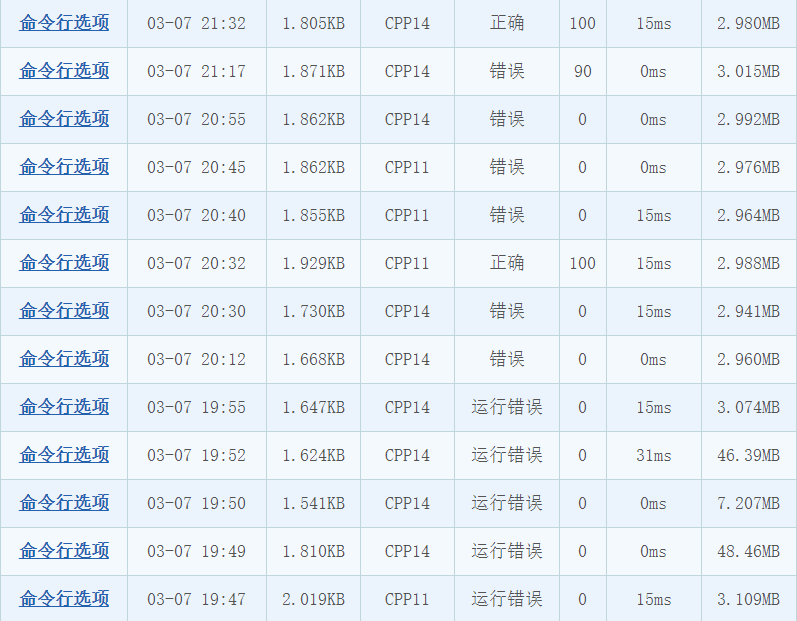# CCF 201403-3 命令行选项

## 样例输入

albw:x
4
ls -a -l -a documents -b
ls
ls -w 10 -x -w 15
ls -a -b -c -d -e -l

## 样例输出

Case 1: -a -l
Case 2:
Case 3: -w 15 -x
Case 4: -a -b

## 代码

#include <bits/stdc++.h>
#define ll long long
#define ull unsigned long long
#define ms(a,b) memset(a,b,sizeof(a))
const int inf=0x3f3f3f3f;
const ll INF=0x3f3f3f3f3f3f3f3f;
const int maxn=1e6+10;
const int mod=1e9+7;
const int maxm=1e3+10;
using namespace std;
string tmp;
vector<string>res;
// 拆分命令行
void get(string s)
{
tmp="";
res.clear();
int l=s.length();
for(int i=0;i<l;i++)
{
if(s[i]==' ')
{
if(tmp=="")
{
tmp="";
continue;
}
string ttmp="";
for(auto j:tmp)
if(j!=' ')
ttmp+=j;
if(ttmp=="")
continue;
res.push_back(ttmp);
tmp="";
i++;
}
tmp+=s[i];
}
res.push_back(tmp);
}
bool check(string s)
{
if(s=='-')
return true;
return false;
}
int main(int argc, char const *argv[])
{
ios::sync_with_stdio(false);
cin.tie(0);
string s,s1;
int n;
cin>>s;
cin.ignore();
int l=s.length();
map<char,int>vis;
for(int i=0;i<l-1;i++)
{
// 带参选项标记为1，不带参选项标记为-1
if(s[i+1]==':')
vis[s[i]]=1,i++;
else vis[s[i]]=-1;
}
vis[s[l-1]]=-1;
cin>>n;
cin.ignore();
int _=0;
while(n--)
{
set<string>st;
getline(cin,s1);
get(s1);
int sz=res.size();
map<string,string>mp;
for(int i=1;i<sz;i++)
{
string ss=res[i];
// 判断是否是参数
int flag=0;
char c=0;
if(ss=='-'&&ss.length()==2)
c=ss,flag=1;
if((flag&&!vis[c])||!(i|flag)||!flag)
break;
if(vis[c]==-1)
{
st.insert(ss);
if(!check(res[i+1]))
break;
}
if(vis[c]==1)
{
if(i==sz-1)
continue;
mp[ss]=res[i+1];
st.insert(ss);
i++;
}
}
cout<<"Case "<<++_<<":";
for(auto i:st)
{
cout<<" "<<i;
if(mp[i]!="")
cout<<" "<<mp[i];
}
cout<<endl;
}
return 0;
}Last modification：April 1st, 2021 at 12:40 pm